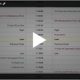# Introduction to Options Theoretical Pricing

Options Education Homepage

Introduction to Theoretical Pricing Models

Option pricing is based on the unknown future outcome for the underlying asset.

If we knew where the market would be at expiration, we could perfectly price every option today. No one knows where the price will be, but we can draw some conclusions using pricing models.

When looking at call options, a higher strike will cost less than a lower strike.

If the underlying asset price has risen dramatically and you chose a higher strike price rather than a lower strike, your payoff will be less because you have foregone the first part of the upward price movement.

For Example

To get an idea of how much the premium should be at each strike, we are going to use a simple model.

Assume an asset is priced at \$100 and has the characteristic of moving one dollar each month (either up or down). In this model, we will assume the price movement repeats every month over the life of the option and the option expiration will occur in four months.

What is the probability for each of the possible price outcomes after four months? In this model there are 16 possible paths that lead to each of the five price outcomes. The probability of each outcome can be calculated by aggregating the paths for each price.The probability of reaching any one price point in this model is the number of paths in that price point divided by the total number of paths.Now that we have the probability for each price point, we can start pricing options with different strike prices. First, you need to know the payoff for each strike price at the defined price level.For example, the 97 call with an underlying price level of 96, would be an out of the money option. The payoff is zero.

At a price level of 98, the 97 call is now in the money, and the payoff is \$1. At 100, the payoff is \$3, at 102 the payoff is \$5 and at 104 the payoff would be \$7.

To find the probability weighted payoff, we multiply the probability for each price point by the payoff amount. The theoretical price for a 97 call would be the sum of the probability weighted payoffs. In this case the sum would be 3.0625.

Continuing the mathematics for each strike price we see the 101 strike has a theoretical price of .4375 and the 103 strike has a theoretical price of.0625.

It should be no surprise the 103 strike has less value than the 101 strike as the probability of it being in the money is much less.

Summary

Traders use proprietary models to determine if the prices in the marketplace are in line with their views. We have shown you a very simple binomial model. Which assumes that the market will move a set amount, either up or down, over each period.

Even the more advanced models still provide only estimates for the option price and are still based on assumptions about the future.

These theoretical pricing models provide options traders the ability to track and measure option prices.

If you have questions or schedule an online review .

Regards,

—————————————————————-

Privacy Notice

Disclosure